# Inadequate proof of Bloch's theorem?

• I
This wasn't the issue I had.

Well, your issue already changed several times. This is the difference in the treatments between Griffith and Bransden/Joachain. Griffith first looks for common eigenstates of the Hamiltonian and the displacement operator for displacement by a single period and then introduces periodic boundary conditions, which is equivalent to looking for all solutions for all displacement operators that correspond to displacement by an integer number of lattice periods. Bransden/Joachain immediately assume periodic boundary conditions at the beginning of their discussion. It is a very different question whether you look for superpositions of functions that are eigenfunctions of one of the displacement operators and the Hamiltonian or superpositions of eigenfunctions of different displacement operators and the Hamiltonian.

There are two values of K for each energy E.

These differ only by sign. Your concern was that these states might result in different $\lambda$. For D(a) as treated by Griffith, you end up with $K=\pm \frac{2\pi}{a}$, which obiously yield the same $\lambda$. This will change when considering a different displacement operator.

Not quite clear what you mean. States that are eigenfunctions of D? States of the same energy?

States that are eigenfunctions of D(a) and the Hamiltonian.

Ok, there may be other ways to get [5.56]. But the book's approach is much easier. It's just one line. And this wasn't my issue. The priority should be on the accurate identifying of the cause of the confusion and addressing it using the simplest tool, without too much unnecessary information. Nonetheless, thank you for your advice.

The approach given in the book is not simpler because what I noted was exactly the approach used in the book.

Gold Member
2022 Award
I think indeed, it's again the enigmatic writing by Griffiths. I don't understand why this book is so successful as it seems to cause so much confusion.

Bloch's theorem is about finding a convenient complete energy eigenbasis. The Hamiltonian is invariant under the unitary translation operators ##\hat{T}(a)=\exp(\mathrm{i} \hat{p} a)##, where ##a## is the periodicity of the (1D) crystal lattice (that generalizes to the 3D case, where instead of ##a## you have the vectors ##\vec{R}## of the Bravais lattice defining the discrete tranlational crystal symmetries of the different classes of possible lattice). You have
$$\hat{H}=\frac{\hat{p}^2}{2m} + V(\hat{x}),$$
and the Hamiltonian obviously has the symmetry iff ##V## is a periodic function of period ##a##, and then
$$\hat{T}(a) \hat{H} \hat{T}^{\dagger}(a)=\frac{\hat{p}^2}{2m} + V(\hat{x}+a \hat{1})=\frac{\hat{p}^2}{2m} + V(\hat{x})=\hat{H} \Rightarrow [\hat{T}(a),\hat{H}]=0.$$
Since unitary operators are normal operators, i.e., ##\hat{T}(a) \hat{T}^{\dagger}(a)=\hat{1}=\hat{T}^{\dagger}(a) \hat{T}(a)## they define a complete set of eigenvectors, and since it commutes with ##\hat{H}## you can find a common eigenbasis of ##\hat{H}## and all ##\hat{T}(a)##. The eigenvalues of ##\hat{T}## are phase factors ##\exp(\mathrm{i} k a)##.

To get a concrete set of ##k##-values you need to impose some boundary condition for the 1D crystal as a whole. Since for bulk properties the exact boundary conditions are of not too much importance, usually one chooses the length of the crystal to be an integer multiple of the primitive period ##a##, ##L=N a## and imposes periodic boundary conditions,
$$\psi(\vec{x}+N a)=\psi(\vec{x}).$$
For the Bloch energy-eigenstates you get for each ##k## being an eigenvector of this kind
$$\exp(\mathrm{i} k N a)=1 \; \Rightarrow \; k N a = 2\pi n, \quad n \in \mathbb{Z}.$$
Thus the possible
$$k=k_n=\frac{2 \pi n}{N}, \quad n \in \mathbb{N}.$$
Of course you can form arbitrary linear combinations of these basis vectors, allow to represent all the allowed Hilbert-space vectors (i.e., the ##\mathrm{L}^2## functions, fulfilling the Born von Karmann boundary conditions), but of course, as you rightfully realized superpositions of basis vectors with different ##k_n##'s are not eigenvectors of ##\hat{T}(a)##.

For the analog 3D case that's known as Born-von Karman boundary conditions, where you have in general a Bravais lattice in position space, defined by a primitive cell (Wigner-Seitz cell) and then a reciprocal lattice in momentum space, which formes again a Bravais lettice with the primitive cell known as the 1st Brillouin zone. If ##\vec{a}_i## are the vectors spanning up the Wigner-Seitz cell the three vectors ##\vec{b}_i## spanning the 1st Brillouin zone are conveniently chosen such that ##\vec{b}_j \cdot \vec{a}_i=2 \pi \delta_{ji}##.

Mentor
the condition that at least one of the functions must be an eigenfunction of ##D## is what places the restriction on ##k#

Actually, on thinking this over, the condition for eigenfunctions of ##H## do also restrict ##k## if we are talking about sines and cosines. For sines and cosines, the eigenvalue equation for ##H## can be written:

$$\left( \frac{\hbar^2 k^2}{2m} + V(x) - E \right) \psi(x) = 0$$

This can only be satisfied if ##V(x)## has the same constant value at every ##x## for which ##\psi(x) \neq 0##. But that means that either ##V(x)## has the same constant value everywhere (which is just the trivial free particle case, not what we're discussing here), or we must have ##\psi(x) = 0## at some values of ##x##, so that ##V(x)## can have a different value at those values of ##x##. But if that happens for any value of ##x##, it must also happen for all other values that differ from that one by an integer multiple of ##a##, because ##V(x)## is periodic with period ##a##. In other words, ##\psi(x)## must have zeros spaced by ##a## (or some integer fraction of ##a##). And for sines and cosines, that is equivalent to the restriction on ##k## that I gave.

I think a similar argument will work for any function ##\psi(x)## that is known to be periodic.

Gold Member
2022 Award
Why should all energy eigensolutions necessarily be periodic? If for some reason an energy eigenvalue is degenerate, i.e., there are Bloch eigenstates with the same ##E## but different ##k_n##, you can form linear combinations being still an energy eigenvector but not periodic with period ##a##.

Not all energy eigensolutions need to obey the symmetry of the underlying dynamics. Even the (then necessarily degenerate) ground states need not obey the symmetry. That's then known as "spontaneous symmetry breaking".

Mentor
Why should all energy eigensolutions necessarily be periodic?

I don't think they need to be in general. In the subthread in question @Happiness had given a specific example of sines and cosines, and I was considering that specific case.

Mentor
if there are Bloch eigenstates with the same ##E## but different ##k_n##

Is there a specific example of such a set of Bloch eigenstates?

Gold Member
2022 Award
For the 1D case, it's hard to imagine... I don't know.

Happiness
it must satisfy k = n ##\pi## / a, where n is an integer and a is the "spacing" in the potential V(x)
This only covers the cases where the eigenvalue of D is ##\lambda=1## (when n is even) and ##\lambda=-1## (when n is odd). For complex values of ##\lambda=e^{iKa}##, ##k\neq\frac{n\pi}{a}##. So there are other values of ##\lambda## that you did not consider, explicitly those complex values, which produce other values of ##k##.
any eigenfunction ##\psi(x)## of D must be periodic with period a
This is only true when ##\lambda=1##. In general, it is the square of the amplitude of any eigenfunction ##\psi_{\lambda}(x)## of D that must be periodic with period a. But not the eigenfunctions themselves. In fact, the eigenfunctions ##\psi_{\lambda}(x)## of D acquire a phase factor ##\lambda=e^{iKa}## when acted upon by D.
Given any value of k (not assuming any restriction on k at the start of the argument), the functions ##\sin(kx)## and ##\cos(kx)## form a basis of a space of functions that all have the same periodicity. Assume that these functions are all eigenstates of H (if anyone of them is, they all are because they all have the same k, and if none of them are, we don't care about this value of k anyway). In order for the conditions needed for Bloch's Theorem to apply at all, at least one of the functions must also be an eigenfunction of D. And if anyone of them is, they all are, since they all have the same periodicity, and periodicity is the only criterion that determines whether a function is an eigenfunction of D.
k is not the only criterion that determines whether a function is an eigenfunction of D: A and B also determine whether a function is an eigenfunction of D, via its effect on the derivative of the function (as the derivative needs to be periodic too), where A and B are as defined in [5.59].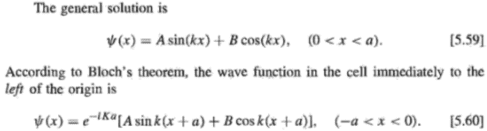The cause of my confusion is that I believe there are eigenstates of H that are not eigenstates of D. This is confirmed by B&J's paragraph following (4.190).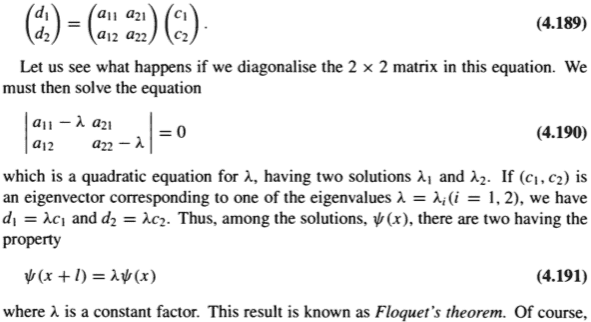Consider the simplest case of ##\psi=A\sin (kx)+B\cos (kx)##. The eigenfunctions of D are given by ##A=\pm iB##. So ##\psi=\sin (kx)## is an eigenfunction of H but not of D. The eigenfunctions of D are ##\psi_{\lambda_1}=e^{ikx}## and ##\psi_{\lambda_2}=e^{-ikx}##. And since they have different eigenvalues, a linear combination of these two eigenfunctions will not be an eigenfunction of D in general, and so does not satisfy Bloch's theorem ##\psi(x+a)=e^{iKa}\psi(x)## even though it is always an eigenfunction of H.

Last edited:
Mentor
The eigenfunctions of D are given by ##A=\pm iB##. So ##\psi=\sin (kx)## is an eigenfunction of ##H## but not of ##D##.

If both the sine and the cosine have the same ##k##, I don't see this. The definition of ##D## is

$$D \psi (x) = \psi(x + a)$$

For the values of ##k## that I gave, we have ##\sin k (x + a) = \sin (kx)##. So ##D \sin(kx) = \sin k (x + a) = \sin (kx)##, and ##\sin (kx)## is an eigenfunction of ##D##. So is ##\cos (kx)## for the same ##k##, and so is any linear combination of them, by the argument I gave earlier.

For other values of ##k##, not meeting the condition I gave earlier that ##k = n \pi / a##, obviously ##\sin(kx)## won't be an eigenfunction of ##D##, but I never claimed it would be.

I understand that if you combine sines and cosines with different values of ##k##, even if each of the ##k## values meets the condition I gave earlier, you won't, in general, get an eigenfunction of ##D##. But you won't, in general, get an eigenfunction of ##H## either.

Mentor
This only covers the cases where the eigenvalue of D is ##\lambda=1## (when n is even) and ##\lambda=-1## (when n is odd).

Actually, it only covers ##\lambda = 1##, since ##\sin (x + n \pi) = \sin x## for any integer ##n##, and similarly for cosines. If I had put ##2 \pi## instead of ##\pi## in the formula just now, that would be different; but I didn't. I agree, though, that I did not cover other possibilities for ##\lambda##. For that more general case, I think it's easier to use exponentials instead of sines and cosines; I'll take a look at that.

Happiness
If both the sine and the cosine have the same ##k##, I don't see this. The definition of ##D## is

$$D \psi (x) = \psi(x + a)$$

For the values of ##k## that I gave, we have ##\sin k (x + a) = \sin (kx)##. So ##D \sin(kx) = \sin k (x + a) = \sin (kx)##, and ##\sin (kx)## is an eigenfunction of ##D##. So is ##\cos (kx)## for the same ##k##, and so is any linear combination of them, by the argument I gave earlier.

For other values of ##k##, not meeting the condition I gave earlier that ##k = n \pi / a##, obviously ##\sin(kx)## won't be an eigenfunction of ##D##, but I never claimed it would be.

I understand that if you combine sines and cosines with different values of ##k##, even if each of the ##k## values meets the condition I gave earlier, you won't, in general, get an eigenfunction of ##D##. But you won't, in general, get an eigenfunction of ##H## either.
$$\psi_{\lambda_1}=B\cos(kx)+iB\sin(kx)=e^{ikx}$$
$$D\psi_{\lambda_1}=e^{ik(x+a)}=e^{ika}e^{ikx}$$
So ##e^{ikx}## is an eigenvector of D with eigenvalue ##e^{ika}##.
$$\psi_{\lambda_2}=B\cos(kx)-iB\sin(kx)=e^{-ikx}$$
$$D\psi_{\lambda_2}=e^{-ik(x+a)}=e^{-ika}e^{-ikx}$$
So ##e^{-ikx}## is an eigenvector of D with eigenvalue ##e^{-ika}##. The k are all the same, so ##\psi_{\lambda_1}## and ##\psi_{\lambda_2}## have the same energy. But ##\frac{\psi_{\lambda_1}-\psi_{\lambda_2}}{2i}=\sin(kx)## is not an eigenfunction of D, even though it is an eigenfunction of H.

(B=1 after normalisation.)

Happiness
Actually, it only covers ##\lambda = 1##, since ##\sin (x + n \pi) = \sin x## for any integer ##n##, and similarly for cosines. If I had put ##2 \pi## instead of ##\pi## in the formula just now, that would be different; but I didn't. I agree, though, that I did not cover other possibilities for ##\lambda##. For that more general case, I think it's easier to use exponentials instead of sines and cosines; I'll take a look at that.
##\sin (x + \pi) = -\sin x##
##\cos (x + \pi) = -\cos x##

Mentor
##\sin (x + \pi) = -\sin x##
##\cos (x + \pi) = -\cos x##

Ah, you're right. <facepalm> So I did need to put ##2 \pi## as the period in my earlier formula (which limits consideration to the case ##\lambda = 1##).

However, with that revised condition on ##k##, the argument I gave in post #44 for ##\sin (kx)## and ##\cos (kx)## being eigenfunctions of ##D## is still valid. In fact the argument can be stated even more simply: ##\sin (kx)## and ##\cos (kx)## are periodic, so if we choose ##k## to have the appropriate relationship to ##a##, they are obviously eigenfunctions of ##D##.

Happiness
Ah, you're right. <facepalm> So I did need to put ##2 \pi## as the period in my earlier formula (which limits consideration to the case ##\lambda = 1##).

However, with that revised condition on ##k##, the argument I gave in post #44 for ##\sin (kx)## and ##\cos (kx)## being eigenfunctions of ##D## is still valid. In fact the argument can be stated even more simply: ##\sin (kx)## and ##\cos (kx)## are periodic, so if we choose ##k## to have the appropriate relationship to ##a##, they are obviously eigenfunctions of ##D##.
Suppose you found that value of ##k## such that ##\sin (kx)## and ##\cos (kx)## are both eigenfunctions of ##D##, then ##\sin (kx)+\cos (kx)## or ##\psi_{\lambda_1}+\psi_{\lambda_2}## will not be an eigenfunction of ##D## in general, because ##\psi_{\lambda_1}## and ##\psi_{\lambda_2}## have different eigenvalues in general. (##\psi_{\lambda_1}+\psi_{\lambda_2}## is always an eigenfunction of ##H##.) It is proven by B&J that ##\lambda_1=\frac{1}{\lambda_2}##.

If you only consider ##\lambda## to be real numbers, then you will mislead yourself into thinking that ##\psi_{\lambda_1}+\psi_{\lambda_2}## is always an eigenfunction of ##D##.

This only covers the cases where the eigenvalue of D is ##\lambda=1## (when n is even) and ##\lambda=-1## (when n is odd). For complex values of ##\lambda=e^{iKa}##, ##k\neq\frac{n\pi}{a}##. So there are other values of ##\lambda## that you did not consider, explicitly those complex values, which produce other values of ##k##.

You still emphasize the wrong point. D(a) is a pedagogical tool to get the intuition right. For a completely periodic potential, you will get a whole family of operators corresponding to D(na), where n is an integer. Any of them represents a symmetry of the system. Any complex $\lambda$ for D(a) will be a real $\lambda$ for D(na) for some n. The reason why you get wavefunctions that do not share the periodicity of the lattice is that all Bloch wave functions in a fully periodic potential need to be simultaneous eigenstates of the Hamiltonian and D(na) for some n, not necessarily of the Hamiltonian and D(a).

Happiness
You still emphasize the wrong point. D(a) is a pedagogical tool to get the intuition right. For a completely periodic potential, you will get a whole family of operators corresponding to D(na), where n is an integer. Any of them represents a symmetry of the system. Any complex $\lambda$ for D(a) will be a real $\lambda$ for D(na) for some n. The reason why you get wavefunctions that do not share the periodicity of the lattice is that all Bloch wave functions in a fully periodic potential need to be simultaneous eigenstates of the Hamiltonian and D(na) for one n, not necessarily of the Hamiltonian and D(a).
What you said is true. But what I'm trying to say is there exist eigenstates of H that are not eigenstates of D.

What you said is true. But what I'm trying to say is there exist eigenstates of H that are not eigenstates of D.

Which D? D(a)? Yes, indeed. I fully agree with that.

Happiness
Which D? D(a)? Yes, indeed. I fully agree with that.
Yes D(a).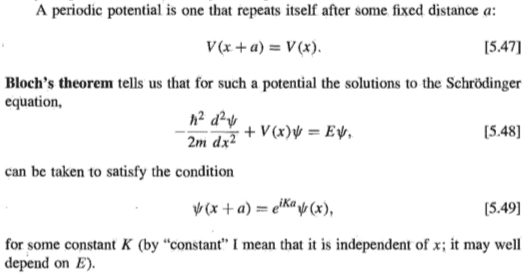This means [5.48] doesn't imply [5.49]. There exist solutions to [5.48] that are not solutions to [5.49]. This is what I have been saying all along:
You could blame it on his [Grifftihs's] phrasing. What Bloch's theorem is saying is that there exist some solutions to the Schrondinger equation [5.48] that satisfy the condition [5.49]. It is not saying all solutions to the Schrondinger equation [5.48] will satisfy the condition [5.49].
in contrast to
No, Bloch's theorem does indeed say that all solutions of [5.48] given that the potential satisfies [5.47] satisfy the condition [5.49].

This means [5.48] doesn't imply [5.49]. There exist solutions to [5.48] that are not solutions to [5.49]. This is what I have been saying:

Huh? No, All solutions to [5.48] are still solutions to [5.49]. [5.49] does not require $\psi$ to be periodic in a. $e^{iKa}$ gives the phase shift between one unit cell and the next one.

Happiness
What you said is true. But what I'm trying to say is there exist eigenstates of H that are not eigenstates of D.
Which D? D(a)? Yes, indeed. I fully agree with that.
What you said here contradicts what you said below
All solutions to [5.48] are still solutions to [5.49].
because [5.48] is an eigenvalue equation for H and [5.49] is an eigenvalue equation for D.

Ok, sloppy language. My fault.
The eigenstates will not share the periodicity of a.
However, D is a unitary operator and not a Hermitian one, so the eigenvalues may be complex and as such do not need to share this periodicity. All solutions of the Hamiltonian are eigenstates of D(a), but they do not necessarily have real eigenvalues. However, all eigenstates will have real eigenvalues for some translation operator.

Mentor
all eigenstates will have real eigenvalues for some translation operator

Just to clarify, I assume you mean all eigenstates of the Hamiltonian will have real eigenvalues for some translation operator.

Just to clarify, I assume you mean all eigenstates of the Hamiltonian will have real eigenvalues for some translation operator.

Sorry, it is getting late. Of course you are right.

Mentor
But ##\frac{\psi_{\lambda_1}-\psi_{\lambda_2}}{2i}=\sin(kx)## is not an eigenfunction of ##D##

It is if ##k## meets the condition I gave earlier, because for those values of ##k##, ##e^{ika} = e^{-ika}##, since ##ka = n \pi## and ##e^{i n \pi} = e^{- i n \pi}##.

Happiness
All solutions of the Hamiltonian are eigenstates of D(a), but they do not necessarily have real eigenvalues.
This is false. Consider the simplest case of ##\psi=\sin(kx)##. It is an eigenstate of H, but not of D. Even with complex eigenvalues, you cannot write ##\sin(kx)## as a complex multiple of ##e^{ikx}## alone or as a complex multiple of ##e^{-ikx}## alone.

Happiness
It is if ##k## meets the condition I gave earlier, because for those values of ##k##, ##e^{ika} = e^{-ika}##, since ##ka = n \pi## and ##e^{i n \pi} = e^{- i n \pi}##.
This is because
If you only consider ##\lambda## to be real numbers, then you will mislead yourself into thinking that ##\psi_{\lambda_1}+\psi_{\lambda_2}## is always an eigenfunction of ##D##.

Mentor
Consider the simplest case of ##\psi=\sin(kx)##. It is an eigenstate of H, but not of D.

Why do you keep making this false statement even after I have shown that it is false?

At the very least, you should acknowledge that there are conditions on ##k## for which ##\sin (kx)## is an eigenstate of ##D##, since I have explicitly shown what those conditions are. And you should also acknowledge that the ##D## you refer to here is what @Cthugha called ##D(a)##, i.e., a specific translation operator out of multiple possible ones.

Happiness
@PeterDonis Do you agree that there exists an allowed value of k such that ##\psi=\sin(kx)## is an eigenstate of H, but not of D?

Mentor
Do you agree that there exists an allowed value of ##k## such that ##\psi=\sin(kx)## is an eigenstate of H, but not of D?

Have you shown one in this discussion?

Happiness
Have you shown one in this discussion?
Ok I didn't show an example explicitly as I thought B&J's paragraph following (4.190) is an adequate proof of what I have been saying.

Anyway, you can find one such example from Griffiths: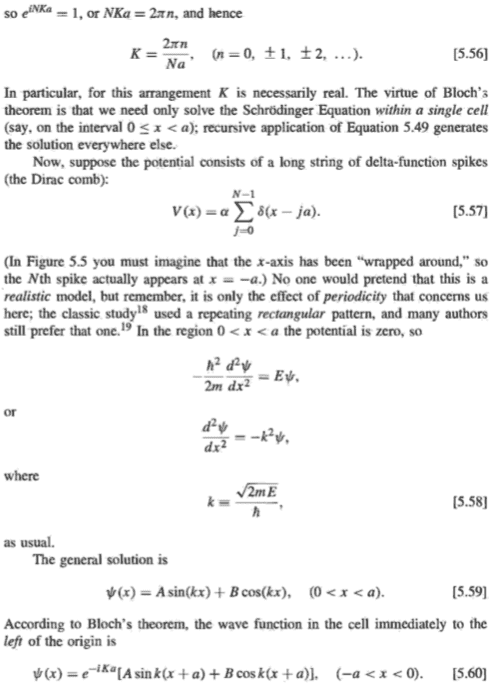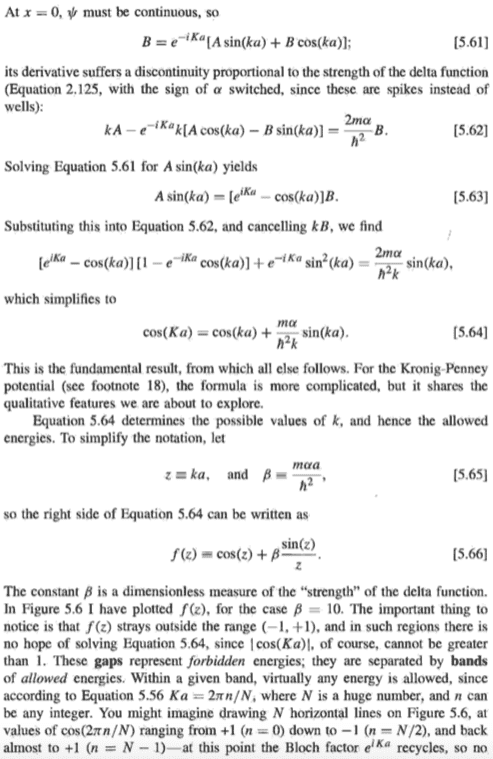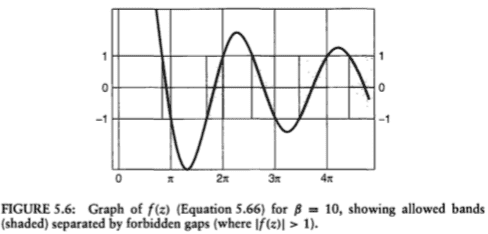For each value of K in [5.56], we solve [5.64] graphically. For each K, we draw a horizontal line in Fig 5.6, and we may get several points of intersection. Each point corresponds to an allowed value of k. (K determines the value on the y axis; k is determined from the x coordinate of the point of intersection.) Each allowed k corresponds to two values of K, as you can see from [5.64] that if K is a solution for a particular k, then -K is also a solution (for the same k). Substitute the big K and small k that you get from a point of intersection into [5.63] to get A in terms of B. Substitute this A into [5.59], and after normalisation, we get a value of A and B that satisfy both [5.48] and [5.49] for an allowed value of k. (Actually, we get two values if you remember to include the case for -K.)

But for this allowed value of k, any A and B would have satisfied [5.48] (since k is the same means energy E is the same), but not [5.49].

Therefore, there exists an allowed value of k such that a solution to [5.48] is not a solution to [5.49].

Therefore, there exists an allowed value of k such that a solution to [5.48] is not a solution to [5.49].

That is not correct. In the band gap region, you arrive at a pair of values of $\lambda$ that is real, but not 1, which corresponds to exponentially growing or decaying modes. Out of these, the decaying mode is usually the physically relevant one.

If you consider the optical equivalent of a periodic potential: a material with periodically structured refractive index, you will find optical band gaps equivalent to the electronic ones. If you shine a light beam in the optical band gap on a (obviously effectively finite size) material structured this way, you will find exactly this exponential decay.

Also, just as a general remark, one should consider that many of the possible superpositions of arbitrary Bloch states will not necessarily be stationary states.

Happiness
Therefore, there exists an allowed value of k such that a solution to [5.48] is not a solution to [5.49].
That is not correct. In the band gap region, you arrive at a pair of values of $\lambda$ that is real, but not 1, which corresponds to exponentially growing or decaying modes. Out of these, the decaying mode is usually the physically relevant one.

What you said seems to contradict Griffiths's statement below: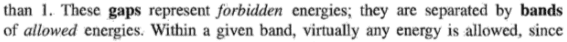Griffiths said states with energies in the gap region are physically impossible, but you said these states are physically relevant.

A definition of "growing mode" and "decaying mode" would be helpful, and also a concise explanation how they are "exponential".

But in any case, my following sentence
Therefore, there exists an allowed value of k such that a solution to [5.48] is not a solution to [5.49].
already set the discussion: we only consider an allowed value of k. But your reply is about disallowed values of k being physically relevant. But I wasn't making any claim about these disallowed values of k!

You seem to be very proselytising in sharing and showing your knowledge in numerous things, like decaying modes, optical materials, modular arithmetic, etc., which may be good in a way, but it may sometimes distract the attention away from the main issue in the discussion.

Simplicity and conciseness are very expensive gifts. (in tribute to Warren Buffett's quote on honesty)

Last edited:
What you said seems to contradict Griffiths's statement below:
View attachment 248117

Griffiths said states with energies in the gap region are physically impossible, but you said these states are physically relevant.

Then you should read up on the definition of forbidden modes. These refer to propagating modes. The "forbidden" modes are a synonym for being non-propagating or evanescent.

A definition of "growing mode" and "decaying mode" would be helpful, and also a concise explanation how they are "exponential".

Well, you already noted that $\lambda_1=\lambda_2^{-1}$ and we had the definition of $\lambda_1=e^{iKl}$ several times already, so it should be trivial to see what happens if the magnitude of $\lambda_1$ and $\lambda_2$ differs from unity.

But in any case, my following sentence

already set the discussion: we only consider an allowed value of k. But your reply is about disallowed values of k being physically relevant. But I wasn't making any claim about these disallowed values of k!

Well, yes, but @PeterDonis already responded to the whole issue beforehand. You then repeated the discussion in post #65 and made it more complicated. This conclusion:

But for this allowed value of k, any A and B would have satisfied [5.48] (since k is the same means energy E is the same), but not [5.49].
Therefore, there exists an allowed value of k such that a solution to [5.48] is not a solution to [5.49].

is not warranted as not any A and B would satisfy e.g. [5.62].
Anyhow, any periodic eigenfunction of the Hamiltonian will at some point match some periodicity of the periodic potential for an infinitely extended system, so it will be an eigenstate of D(na) with one of the eigenvalues equal to 1 for some n. This also determines the eigenvalues for D(a).

Simplicity and conciseness are very expensive gifts. (in tribute to Warren Buffett's quote on honesty)

Over several years of teaching physics I learned that giving answers that match the simplicity and conciseness of the question is the best approach.

Happiness
This conclusion:

is not warranted as not any A and B would satisfy e.g. [5.62].
[5.62] is used to search for common eigenstates of H and D, since it is obtained from the eigenvalue equations of H [5.48] and of D [5.49]. Therefore, not any A and B would satisfy e.g. [5.62]. Isn't it true that any A and B would satisfy [5.48] for a particular E since [5.59] is its general solution?

You then repeated the discussion in post #65 and made it more complicated.
The post is a response to PeterDonis's request for an example. It is not complicated. You may need some time to read it, yes, but it's not complicated.

Last edited:
The purpose of [5.62] is not to find common eigenstates, but to find the solutions to the Hamiltonian. The idea is to solve it in a single unit cell $0\leq x<a$. [5.58] gives the free particle Hamiltonian in the absence of a potential for $0< x<a$. [5.59] is its general solution. However you still need the value for 0. [5.59] does not tell you anything about this, so [5.59] is not the general solution to [5.48]. Usually you would have to solve the full Schrödinger equation including the potential at every point. As in this case the potential is 0 everywhere, except for 0 and a, you get away with the easier procedure of taking the general potential-free case for $0< x<a$ and adding the influence of the potential as a boundary value problem for 0 and a.
You also see from [5.57] that $\alpha$ corresponds to the magnitude of the potential, so the solution to the full problem at hand must depend on it, which [5.59] does not. Accordingly [5.59] cannot the general solution to [5.48].